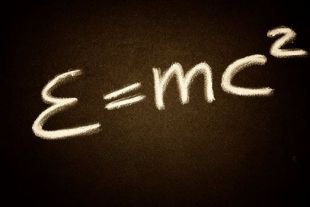Search IntMath
Close

450+ Math Lessons written by Math Professors and Teachers

5 Million+ Students Helped Each Year

1200+ Articles Written by Math Educators and Enthusiasts

Simplifying and Teaching Math for Over 23 Years

# 2. Multiplication of Algebraic ExpressionsEinstein's famous equation involves multiplying algebraic terms

When we multiply algebraic expressions, we need to remember the Index Laws from the Numbers chapter.

Let's see how algebra multiplication works with a series of examples.

## Example 1

Multiply x3(x4 + 5a)

We expand this out using the distributive law:

x3(x4 + 5a)

= x7 + 5ax3

We cannot simplify this answer any further. We present our answer in alphabetical order since it makes it easier to read when the problems become more involved.

## Example 2

Multiply (x + 5)(a − 6)

We multiply this out as follows. We take each term of the first bracket and multiply them by the second bracket. Then we expand out the result.

(x + 5)(a − 6)

= x(a − 6) + 5(a − 6)

= ax − 6x + 5a − 30

We cannot do any more with this answer. There are no like terms, so we cannot simplify it in any way.

## Example 3

Multiply (2x + 3)(x2x − 5)

We take the 2 terms of the first bracket and multiply both of them by the second bracket.

(2x + 3)(x2x − 5)

= (2x)(x2x − 5) + (3)(x2x − 5)

= (2x3 − 2x2 − 10x) + (3x2 − 3x − 15)

= 2x3 + x2 − 13x − 15

This time we could collect together some like terms. There was:

−2x2 + 3x2 = x2

and

−10x − 3x = −13x

## Example 4

Multiply (x - 3)^2

(x − 3)2

= (x − 3)(x − 3)

= x(x − 3) − 3(x − 3)

= x2 − 3x − 3x + 9

= x2 − 6x + 9

## Important Note:

(x - 3)^2

is NOT equal to

x^2 - 9

Please take note of this! Many students confuse this idea and it's not surprising because sometimes the way we write math is not consistent. (See also Towards more meaningful math notation for a discussion on this issue.)

## Example 5

Simplify 5x[−4 + 10(xy)] + 7x

5x[−4 + 10(xy)] + 7x

= 5x[−4 + 10x − 10y] + 7x

= −20x + 50x2 − 50xy + 7x

= −13x + 50x2 − 50xy

## Example 6

Simplify (p − 1)(c − 1) + 2

p(c − 1) − 1(c − 1) + 2

= pcpc + 1 + 2

= pcpc + 3

## Problem SolverThis tool combines the power of mathematical computation engine that excels at solving mathematical formulas with the power of GPT large language models to parse and generate natural language. This creates math problem solver thats more accurate than ChatGPT, more flexible than a calculator, and faster answers than a human tutor. Learn More.